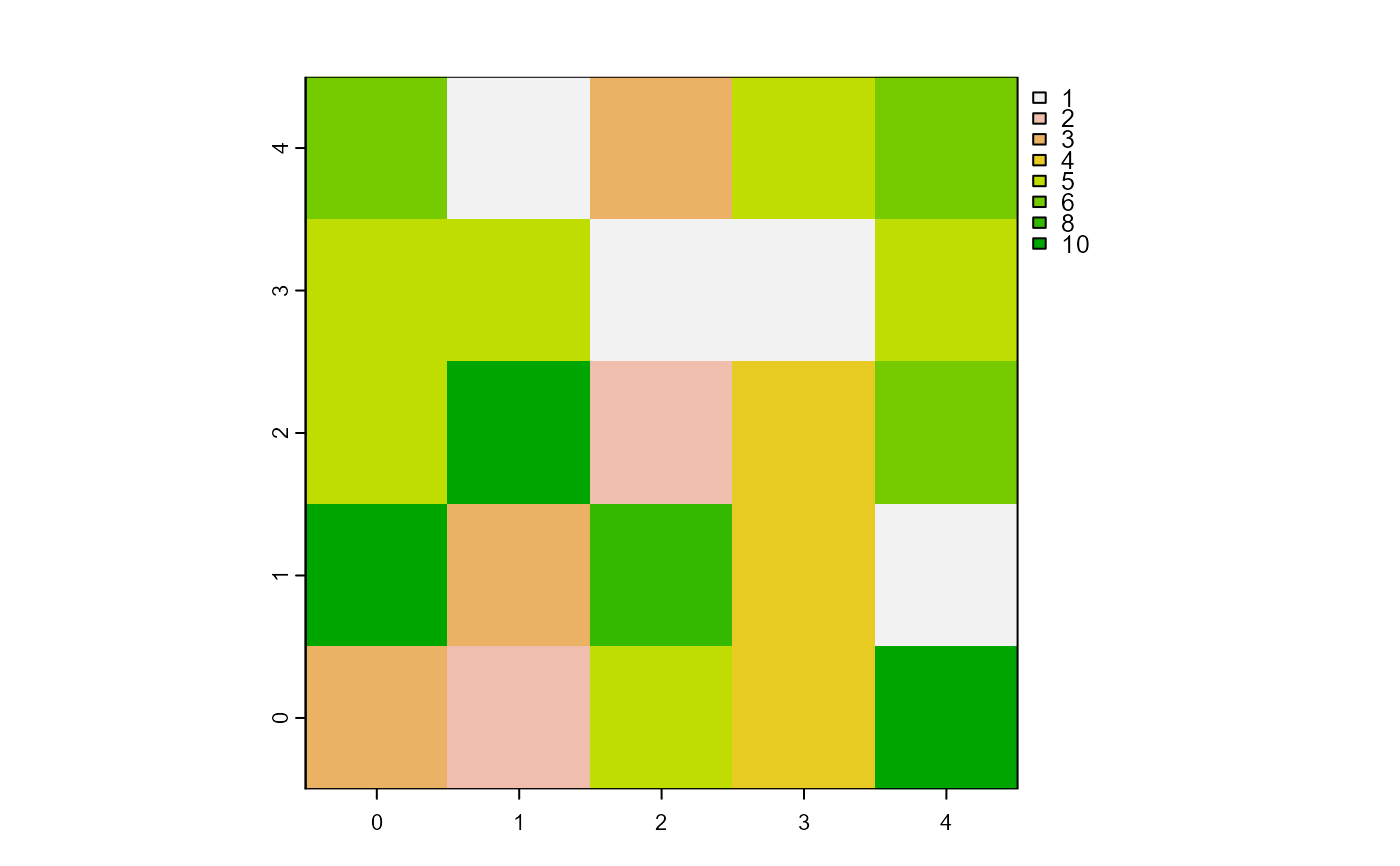Report the n patches or turtles among agents which have their variable among the minimum values.

minNof(agents, n, world, var)

# S4 method for matrix,numeric,worldMatrix,missing
minNof(agents, n, world)

# S4 method for matrix,numeric,worldArray,character
minNof(agents, n, world, var)

# S4 method for agentMatrix,numeric,missing,character
minNof(agents, n, var)

## Arguments

agents

Matrix (ncol = 2) with the first column pxcor and the second column pycor representing the patches coordinates, or

AgentMatrix object representing the moving agents.

n

Integer.

world

WorldMatrix or worldArray object.

var

Character. The name of the selected agents variable. If agents are patches and the world is a worldMatrix object, var must not be provided. If agents are patches and the world is a worldArray object, var is the name of the layer to use to define the patches values. If agents are turtles, var is one of the turtles' variable and can be equal to xcor, ycor, any of the variables created when turtles were created, as well as any variable created using turtlesOwn().

## Value

Matrix (ncol = 2, nrow = n) with the first column pxcor and the second column pycor representing the coordinates of the npatches among the agents which have their variable values among the minimum values among the agents, or AgentMatrix of length n representing the turtles among the agents which have their var values among the minimum values among the agents.

## Details

world must not be provided if agents are turtles.

If there is a tie that would make the number of returned patches or turtles larger than n, it is broken randomly.

Sarah Bauduin

## Examples

# Patches
w1 <- createWorld(minPxcor = 0, maxPxcor = 4, minPycor = 0, maxPycor = 4,
data = sample(1:10, size = 25, replace = TRUE))
plot(w1)p1 <- minNof(agents = patches(w1), n = 6, world = w1)

# Turtles
t1 <- createTurtles(n = 10, coords = randomXYcor(w1, n = 10),
heading = sample(1:5, size = 10, replace= TRUE))
t2 <- minNof(agents = t1, n = 5, var = "heading")